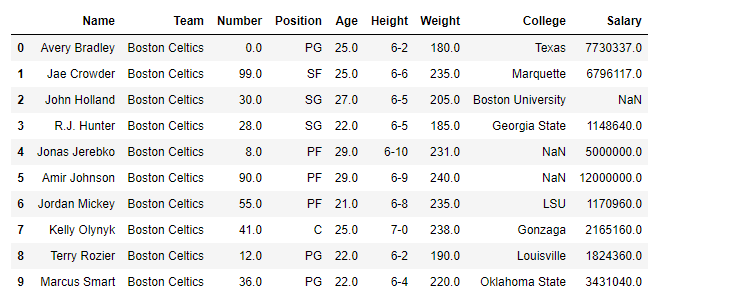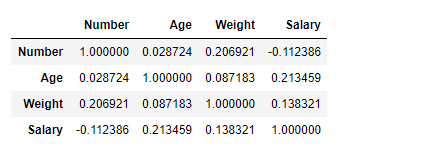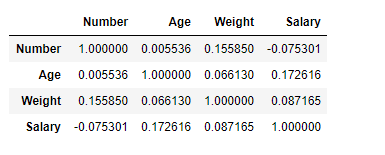# Python | Pandas dataframe.corr()

• Difficulty Level : Basic
• Last Updated : 22 Apr, 2020

Python is a great language for doing data analysis, primarily because of the fantastic ecosystem of data-centric python packages. Pandas is one of those packages and makes importing and analyzing data much easier.

Pandas` dataframe.corr()` is used to find the pairwise correlation of all columns in the dataframe. Any `na` values are automatically excluded. For any non-numeric data type columns in the dataframe it is ignored.

Syntax: DataFrame.corr(self, method=’pearson’, min_periods=1)

Parameters:
method :
`pearson `: standard correlation coefficient
`kendall `: Kendall Tau correlation coefficient
`spearman `: Spearman rank correlation
min_periods : Minimum number of observations required per pair of columns to have a valid result. Currently only available for pearson and spearman correlation

Returns: count :y : DataFrame

Note: The correlation of a variable with itself is 1.

Example #1: Use `corr()` function to find the correlation among the columns in the dataframe using ‘Pearson’ method.

 `# importing pandas as pd``import` `pandas as pd`` ` `# Making data frame from the csv file``df ``=` `pd.read_csv(``"nba.csv"``)`` ` `# Printing the first 10 rows of the data frame for visualization``df[:``10``]`Now use `corr()` function to find the correlation among the columns. We are only having four numeric columns in the dataframe.

 `# To find the correlation among``# the columns using pearson method``df.corr(method ``=``'pearson'``)`

Output :The output dataframe can be interpreted as for any cell, row variable correlation with the column variable is the value of the cell. As mentioned earlier, that the correlation of a variable with itself is 1. For that reason all the diagonal values are 1.00

Example #2: Use `corr()` function to find the correlation among the columns in the dataframe using ‘kendall’ method.

 `# importing pandas as pd``import` `pandas as pd`` ` `# Making data frame from the csv file``df ``=` `pd.read_csv(``"nba.csv"``)`` ` `# To find the correlation among``# the columns using kendall method``df.corr(method ``=``'kendall'``)`

Output :The output dataframe can be interpreted as for any cell, row variable correlation with the column variable is the value of the cell. As mentioned earlier, that the correlation of a variable with itself is 1. For that reason all the diagonal values are 1.00.

My Personal Notes arrow_drop_up